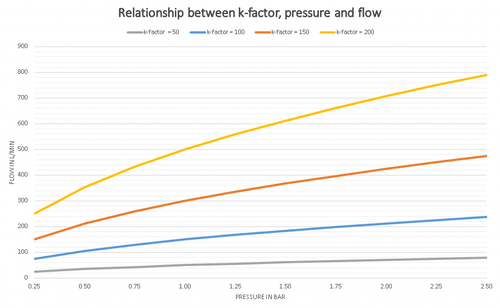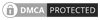## K-Factor formula for fire sprinklers

In this article, we are looking at the flow of water through an orifice and we will define the orifice as an opening (with closed perimeter) in an element of a flow system. For us this orifice will be a fire sprinkler head or water mist nozzle in a fire protection system, we can use the k-factor formula for almost any rounded orifice.

In 1644 an Italian physicist Torricelli (a pupil of Galileo and also invented the barometer) discovered that the flow though an orifice varied to the root of the pressure and later determined the following basic relationship:

Q = AV

when:
Q = flow from the orifice
A = cross-sectional area of the orifice
V = velocity

This has to lead to the accepted theorem for flow though a round orifice:

Q = A√(2gh)

The formula above is theoretical and once we take into account the effects of friction, turbulence and the contraction of the water stream the formula can be simplified to what we know as the k-factor formula for fire protection system by reducing its complexity to a single constant "k".

The K factor formula for fire protection

When we start any hydraulic calculation for a water based fire protection systems such as a fire sprinklers, water mist systems the k-factor formula is the first which we will need to use and as it is so fundamental all fire protection engineers must have a good understanding of how it works. In its most common form, the formula allows us to calculate the discharge flow from the nozzle (fire sprinkler, water mist or a deluge nozzle) if we are given the head pressure and k-factor, we can also calculate the k-factor or the pressure required with this formula.

The discharge from a sprinkler head or water mist nozzle can be calculated from the formula below:

q = kp0.5

when:
q = flow
k = nozzle discharge coefficient or k-factor for head
p = pressure

We can rewrite the formula to give us the k-factor as below:

k = q / p0.5

or the pressure as below:

p = ( q / k )2

The units which we use are important and much not be mixed. you much also be very cautious with the k factor and ensure that you get the correct value for a metric or imperial calculation, the units for both are given below:

for metric calculations:
p = pressure in Bar
q = flow in litre per minute
k = discharge constant Lpm/Bar0.5

and for imperial calculation:
p = pressure in psi
q = flow in gpm
k = discharge constant gpm/psi0.5

We can also use K-factors for many other applications in fire hydraulics such as flow from a fire hydrant, wet riser outlet, hose reel or foam monitor. In fact, the list is almost endless and this is why it is important to be familiar with the above formulas. Often K-factors are given as an imperial value in gpm/psi½ this value cannot be entered into FHC without first converting to its metric equivalent Lpm/bar½. To convert gpm/psi½ to Lpm/bar½ we need to multiply by 14.4 (Approximate) Example: A sprinkler head has a discharge coefficient of 4.2 gpm/psi½ what is its metric equivalent valve. 4.2 x 14.4 = 60.48 Lpm/bar½.We only need to use K-factors to one decimal place so 60.48 becomes 60.5 Lpm/bar½

### Typical k factor values for sprinkler and water mist heads

For many standard type of sprinkler the design standards such as EN 12845 & NFPA 13 specify the standard k-factors and minimum pressure which should be used for different Hazard classifications and design densities. For all other types of sprinkler heads the manufactures data sheet should be referred to for the k-factor and minimum head pressure.

 Hazard Class Design Density (mm/min) K-factor Minimum Pressure (Bar) 1 Light Hazard 2.25 57 0.70 2 Ordinary Hazard 5.00 80 0.50

As a designer, you must check the k-factor value for the nozzle or head manufacturer and ensure its application is correct. You should also seek guidance from the design standard which is applicable.

### The relationship between the k-factor, pressure and flow

The graph below shows the relationship between the k-factor, pressure and flow.  You can clearly see from this that for same pressure with a high k-factor nozzle the flow from the head or nozzle increases.  We can sometimes use this to our advantage by selecting the correct sprinkler head k-factor to provide the design density required with the minimum energy requirement (water pressure).As an example if we have the pressure of 1.50 Bar and a k-factor of 50 the flow rate would be 61.20 L/min for a k-factor of 100 it would be 122.50 L/min and with a k-factor of 150, the flow rate would be 183.70 L/min.  You can see that the flow has insecure by about 61 L/min each time we changed the k-factor by 50, this is because the pressure has remained the same at 1.50 Bar and if you look at the k-factor formula above the pressure is squared which will give us 1.225, this is then multiplied by the head k-factor in this case 50, 100 and 150 in are example. As the k-factor has increased by 50 each time the increase in flow has also increased by the same amount each time.

If you like this article then you may also be interested in our online K-factor calculator.Canute LLP
15 Queen Square
Leeds, West Yorkshire
United Kingdom LS2 8AJ

t: +44 (0) 113 328 0350
e: info@canutesoft.com

 Copyright © 2021, Canute LLPRegistered in England & Wales, Partnership No. OC305985